# 2. 解法

LIS问题基本上都是用动态规划（Dynamic Programming, DP），时间复杂度$O(N^2)$；优化后时间复杂度可以达到$O(NlogN)$。

## 2.1. 朴素DP

• 如果$E_k$的长和宽都严格大于$E_i$，就能形成一个长度为$L_i+1$的信封链。
• 否则，就只能靠$E_k$生成长度为$1$的信封链了。

## 2.2. 优化DP

• 如果$E_{min}$能放进$E_k$里面，则$L_{min}+1$就是$L_k$的一个候选值，没必要再比较另外两个信封了（反正就算能放进，也是同一个候选值）。
• 如果$E_{min}$也无法放进$E_k$，那么另外两个“较大的信封”自然也无法放进$E_k$，所以也没必要比较了。

• 长度1的信封链的最小末端信封
• 长度2的信封链的最小末端信封
• ……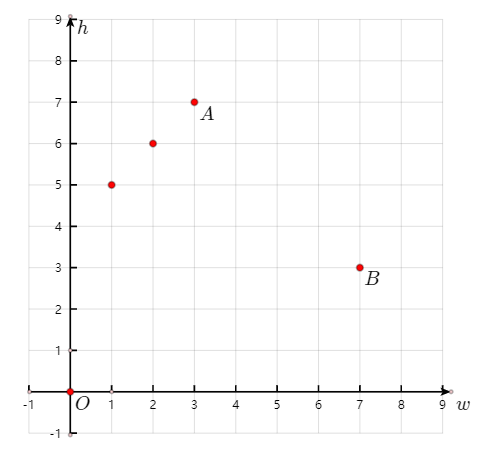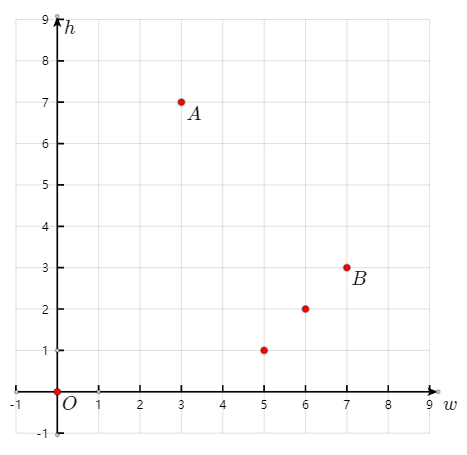### 2.2.1. 佯谬：何为最小？

• (1,1), (2,3)
• (1,1), (3,2)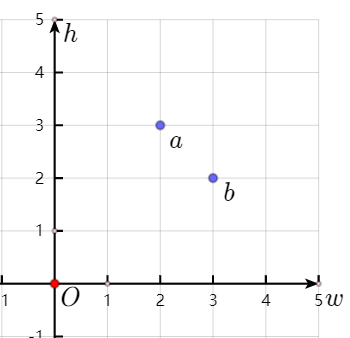• 如果“长度2的信封链的最小末端信封”是$(2,3)$，那么$E_4$就能包裹住它，从而$L_4=L_3+1=2+1=3$。
• 而如果“长度2的信封链的最小末端信封”是$(3,2)$，很明显$E_4$不能包裹住它，于是$E_4$就只能接在$E_1=(1,1)$的后面，形成$L_4=2$的结果。

### 2.2.2. 佯谬的解决

• 在最开始排序的时候，对于每个信封$(w_i, h_i)$，优先按照$w$从小到大排序；但当$w$相同的时候，按照$h$从大到小排序。
• 两个长度相同的信封链，其末端信封的“大小”以末端信封的$h$值为准。如果$h$相同，则哪一个都行。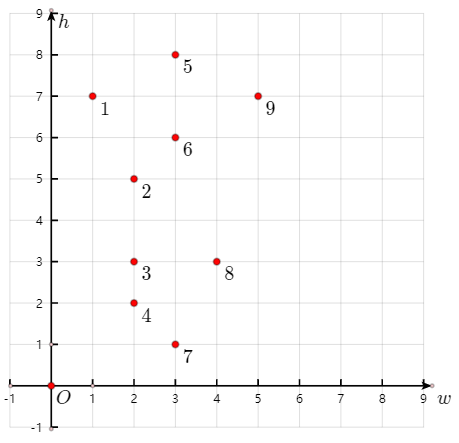### 2.2.3. 佯谬解决方案正确性的证明

• 如果两个信封中$w$和$h$值都完全相同，则选哪一个信封都没区别。
• 如果两个信封中$w$和$h$值之一对应相同，则信封的大小就以另一个不相同的参数的大小关系为准。
• 如果两个信封中$w$和$h$值的大小关系严格一致（比如$w_a<w_b$且$h_a<h_b$），那么大小关系就确定了。
• 下面讨论$w_a<w_b$且$h_a>h_b$的情形。

• 要么$w_k=w_b>w_a$且$h_k<h_b$：由于$h_b<h_a$所以$h_k<h_a$，这时$E_k$既不能包裹$E_a$又不能包裹$E_b$。
• 要么$w_k>w_b>w_a$。此时关键就是比较$h$值。由于$h_b$还比$h_a$小，$h_k$只需要和$h_b$比较就行。所以此时用$E_b$作为“最小末端信封”不会错过最长信封链。

## 2.3. 再进一步优化DP

• 长度1的信封链的中$h$最小的末端信封$E_{end}^1$。
• 长度2的信封链的中$h$最小的末端信封$E_{end}^2$。
• ……
• 长度$l$的信封链的中$h$最小的末端信封$E_{end}^l$。（目前最长的信封链的长度是$l$）

### 2.3.3. 可视化证明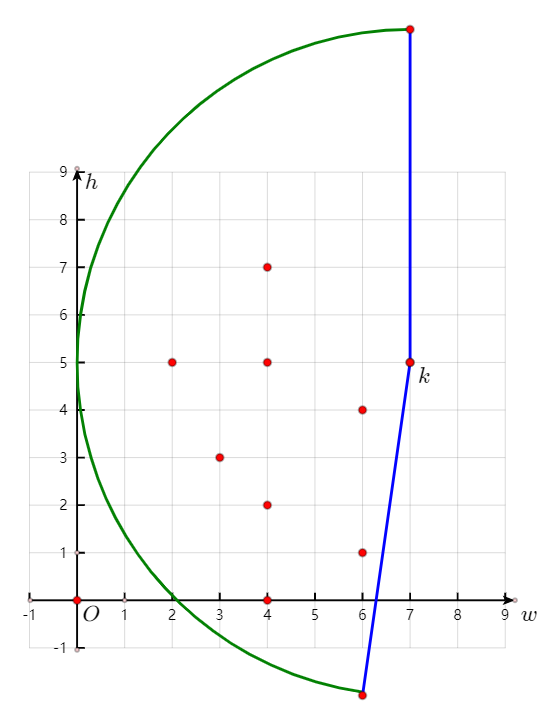## 2.5. 算法

  1 2 3 4 5 6 7 8 9 10 11 12 13 14 15 16 17 18 19 20 21 22 23 24 25 26 27 28 29 30 31 32 33 34 35 36  class Solution { static bool compareEnvelopes(vector &a, vector &b) { if (a == b) { return a > b; } return a < b; } public: int maxEnvelopes(vector>& envelopes) { sort(envelopes.begin(), envelopes.end(), compareEnvelopes); vector minHeight(1, INT_MIN); // [i]的值表示所有长度i的信封链的末端信封的最小h值。无意义。 for (int i = 0; i < envelopes.size(); i++) { auto &now = envelopes[i]; int targetPos = search(minHeight, now); if (targetPos + 1 >= minHeight.size()) { minHeight.push_back(now); } else { minHeight[targetPos + 1] = min(minHeight[targetPos + 1], now); } } return minHeight.size() - 1; } int search(vector &minHeight, int nowHeight) { int begin = 0; int end = minHeight.size(); while (begin + 1 < end) { int mid = (begin + end) / 2; if (minHeight[mid] >= nowHeight) { end = mid; } else { begin = mid; } } return begin; } };